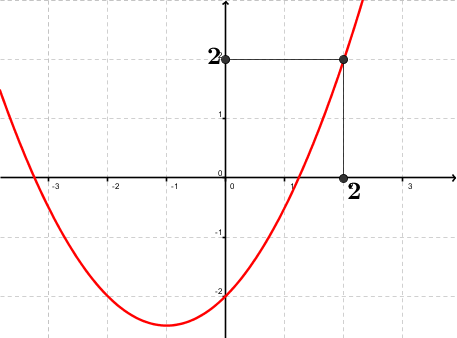Math Calculators, Lessons and Formulas

It is time to solve your math problem

mathportal.org
• Polynomials
• Polynomials basics
• Evaluating polynomials

# Evaluating polynomials

ans:
syntax error
C
DEL
ANS
±
(
)
÷
×
7
8
9
4
5
6
+
1
2
3
=
0
.
auto next question
calculator
•  Question 1: 1 pts Evaluate $x + 2$ for $x = -3$
 5 -1 1 4
•  Question 2: 1 pts Evaluate $-x - 4$ at $x = -4$
 0 8 -8 4
•  Question 3: 1 pts evaluate $x^{17}+4x^{12}-5x^2-x+1$ for $x = 0$
 1 11 111 1111
•  Question 4: 2 pts evaluate $x^{53}+x^{28}+1$ at $x =-1$
 -1 0 1 2
•  Question 5: 2 pts It is easier to evaluate a polynomial before like terms have been combined than after.
•  Question 6: 2 pts if $p(x) = x^2+x+2$, than $p(-2) = 0$
•  Question 7: 2 pts Chose correct answer using the graph of the polynomial $p(x)$ (whose graph is shown in the figure).p(1)=2 p(-2)>0 p(3)>2 p(-4)<0
•  Question 8: 2 pts evaluate $a^3b^2-a^2b+ab^3+b+14$ at $a=0$, $b=-24$
 -10 -11 -12 -13
•  Question 9: 2 pts If $p(a,b) = a^2b + ab$ than $f(-1,-1)=0$
•  Question 10: 3 pts evaluate $a^2b-b^2a$ at $a=121$, $b=121$
 121 11 0 -121
•  Question 11: 3 pts If $p(a,b)=-2a^3b+2$ than $p(-1,x) =$
 $p(-1,x)=12$ $p(-1,x)=2b+2$ $p(-1,x)= 2x+2$ $p(-1,x)=2x^3+2$
•  Question 12: 3 pts if $p(a^3,b) = ab-b$ than
 $p(8,1)=1$ $p(8,1)=2$ $p(8,1)=3$ $p(8,1)=4$
•  Question 13: 3 pts If $p(x)=(x+1)(3-x)(x-45)^2$ than $p(-31)<0$